Practice the problems of Math in Focus Grade 3 Workbook Answer Key Chapter 10 Practice 3 Addition to score better marks in the exam.

Change dollars and cents to cents. Then add.

Example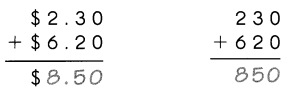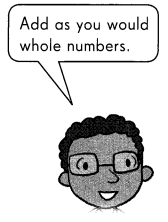Question 1.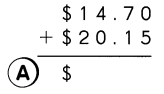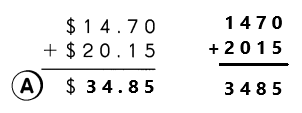Explanation:
First we have to change dollars and cents to cents. We know that 1 us dollar is equal to 100 us cents.
Convert $14.70 into 1470¢ and$20.15 into 2015¢.
Add 1470¢ with 2015¢ the sum is 3485¢.
The cents 3485¢ in dollar form as $34.85. Question 2.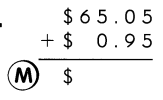Answer: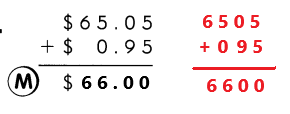Explanation: First we have to change dollars and cents to cents. We know that 1 us dollar is equal to 100 us cents. Convert$65.05 into 6505¢ and $0.95 into 95¢. Add 6505¢ with 95¢ the sum is 6600¢. The cents 6600¢ in dollar form as$66.00.

Question 3.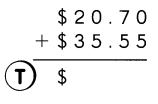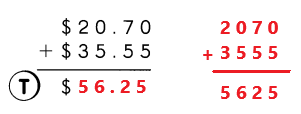Explanation:
First we have to change dollars and cents to cents. We know that 1 us dollar is equal to 100 us cents.
Convert $20.70 into 2070¢ and$35.55 into 3555¢.
Add 2070¢ with 3555¢ the sum is 5625¢.
The cents 5625¢ in dollar form as $56.25. Question 4.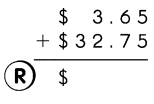Answer: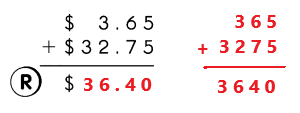Explanation: First we have to change dollars and cents to cents. We know that 1 us dollar is equal to 100 us cents. Convert$3.65 into 365¢ and $32.75 into 3275¢. Add 365¢ with 3275¢ the sum is 3640¢. The cents 3640¢ in dollar form as$36.40.

Question 5.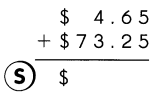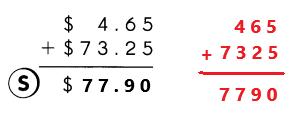Explanation:
First we have to change dollars and cents to cents. We know that 1 us dollar is equal to 100 us cents.
Convert $4.65 into 465¢ and$73.25 into 7325¢.
Add 465¢ with 7325¢ the sum is 7790¢.
The cents 7790¢ in dollar form as $77.90. Question 6.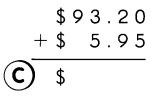Answer: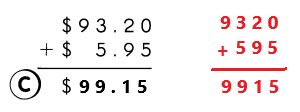Explanation: First we have to change dollars and cents to cents. We know that 1 us dollar is equal to 100 us cents. Convert$93.20 into 9320¢ and $5.95 into 595¢. Add 9320¢ with 595¢ the sum is 9915¢. The cents 9915¢ in dollar form as$99.15.

Question 7.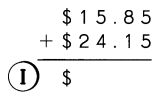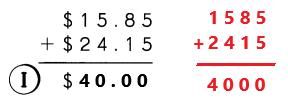Explanation:
First we have to change dollars and cents to cents. We know that 1 us dollar is equal to 100 us cents.
Convert $15.85 into 1585¢ and$24.15 into 2415¢.
Add 1585¢ with 2415¢ the sum is 4000¢.
The cents 4000¢ in dollar form as $40.00. Question 8.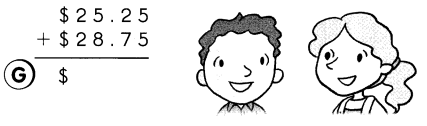Answer: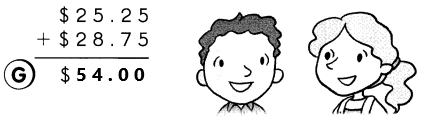Explanation: First we have to change dollars and cents to cents. We know that 1 us dollar is equal to 100 us cents. Convert$25.25 into 2525¢ and $28.75 into 2875¢. Add 2525¢ with 2875¢ the sum is 5400¢. The cents 5400¢ in dollar form as$54.00.

Write the letter that matches each answer to find out.

Question 9.
How do you thank a person in Spanish?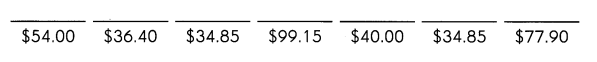Look at the picture. Write the prices, and then add.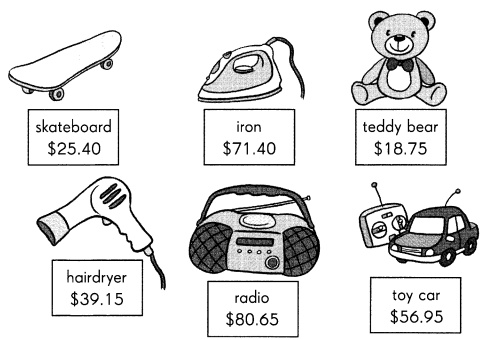Example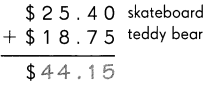Question 10.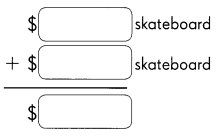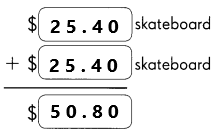Explanation:
In the above image we can observe the price of the skateboard is $25.40. Here both are skate boards. Add$25.40 with $25.40 the sum is$50.80.

Question 11.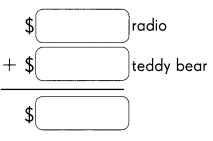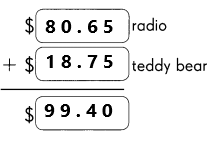In the above image we can observe the price of the radio is $80.65 and the price of the teddy bear is$18.75. Add $80.65 with$18.75 the sum is $99.40. Question 12.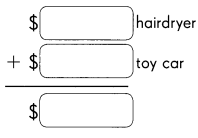Answer: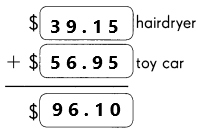Explanation: In the above image we can observe the price of the hairdryer is$39.15 and the price of the toy car is $56.95. Add$39.15 with $56.95 the sum is$96.10.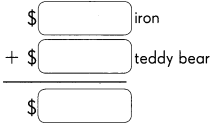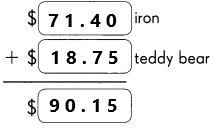In the above image we can observe the price of the iron is $71.40 and the price of the teddy bear is$18.75. Add $71.40 with$18.75 the sum is $90.15. Question 14.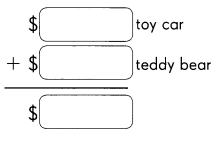Answer: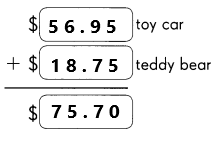Explanation: In the above image we can observe the price of the toy car is$56.95 and the price of the teddy bear is $18.75. Add$56.95 with $18.75 the sum is$75.70.